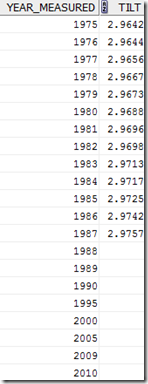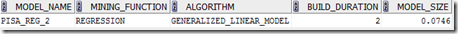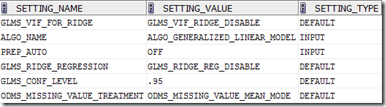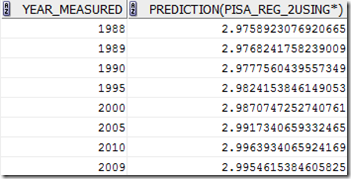## Wednesday, June 20, 2012

### Part 2 of the Leaning Tower of Pisa problem in ODM

In previous post I gave the details of how you can use Regression in Oracle Data Miner to predict/forecast the lean of the tower in future years. This was based on building a regression model in ODM using the known lean/tilt of the tower for a range of years.

In this post I will show you how you can do the same tasks using the Oracle Data Miner functions in SQL and PL/SQL.

Step 1 – Create the table and data

The easiest way to do this is to make a copy of the PISA table we created in the previous blog post. If you haven’t completed this, then go to the blog post and complete step 1 and step 2.

create table PISA_2
as select * from PISA;Step 2 – Create the ODM Settings table

We need to create a ‘settings’ table before we can use the ODM API’s in PL/SQL. The purpose of this table is to store all the configuration parameters needed for the algorithm to work. In our case we only need to set two parameters.

BEGIN
delete from pisa_2_settings;
INSERT INTO PISA_2_settings (setting_name, setting_value) VALUES
(dbms_data_mining.algo_name, dbms_data_mining.ALGO_GENERALIZED_LINEAR_MODEL);
INSERT INTO PISA_2_settings (setting_name, setting_value) VALUES
(dbms_data_mining.prep_auto,dbms_data_mining.prep_auto_off );
COMMIT;
END;

Step 3 – Build the Regression Model

To build the regression model we need to use the CREATE_MODEL function that is part of the DBMS_DATA_MINING package. When calling this function we need to pass in the name of the model, the algorithm to use, the source data, the setting table and the target column we are interested in.

BEGIN
DBMS_DATA_MINING.CREATE_MODEL(
model_name          => 'PISA_REG_2',
mining_function     => dbms_data_mining.regression,
data_table_name     => 'pisa_2_build_v',
case_id_column_name => null,
target_column_name  => 'tilt',
settings_table_name => 'pisa_2_settings');
END;

After this we should have our regression model.

Step 4 – Query the Regression Model details

To find out what was produced as in the previous step we can query the data dictionary.

SELECT model_name,
mining_function,
algorithm,
build_duration,
model_size
from USER_MINING_MODELS
where model_name like 'P%';select setting_name,
setting_value,
setting_type
from all_mining_model_settings
where model_name like 'P%';Step 5 – Apply the Regression Model to new data

Our final step would be to apply it to our new data i.e. the years that we want to know what the lean/tilt would be.

SELECT year_measured, prediction(pisa_reg_2 using *)
FROM   pisa_2_apply_v;